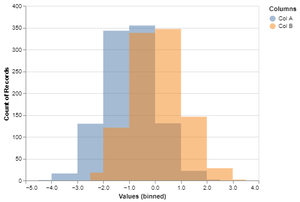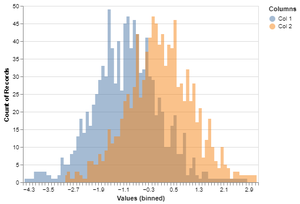Open in App
Not now

# How to Make Overlapping Histograms in Python with Altair?

• Last Updated : 02 Dec, 2020

Prerequisite: Altair

A histogram represents data provided during a sort of some groups. It is an accurate method for the graphical representation of numerical data distribution. It is a kind of bar plot where the X-axis represents the bin ranges while the Y-axis gives information about frequency.

Using Altair, we can make overlapping histograms or layers histograms from data that is in either wide form or long tidy form.

## Procedure

This will common to both forms:

• Import Libraries
• Import or create data.
• Make the data long/wide according to the method.
• Plot the histograms.

Method 1: Tidy form

• To make histogram with Altair, we are using mark_area() function. Here we specify transparency level with opacity argument and therefore the key argument that creates histogram is interpolate=’step’. Without that the histogram would appear as area chart from Altair.
• Then we specify the variables and therefore the number of bins. To differentiate between different plots alt.Color() is employed with the specific variable like multiple histograms.

Example :

## Python3

 `# importing libraries``import` `pandas as pd``import` `altair as alt``import` `numpy as np`` ` ` ` `np.random.seed(``42``)`` ` `# creating data``df ``=` `pd.DataFrame({``'Col A'``: np.random.normal(``-``1``, ``1``, ``1000``),``                   ``'Col B'``: np.random.normal(``0``, ``1``, ``1000``)})`` ` `# Overlapping Histograms``alt.Chart(pd.melt(df,``                  ``id_vars``=``df.index.name,``                  ``value_vars``=``df.columns,``                  ``var_name``=``'Columns'``,``                  ``value_name``=``'Values'``)``          ``).mark_area(opacity``=``0.5``,``                      ``interpolate``=``'step'``                      ``).encode(``    ``alt.X(``'Values'``, ``bin``=``alt.``Bin``(maxbins``=``10``)),``    ``alt.Y(``'count()'``, stack``=``None``),``    ``alt.Color(``'Columns'``)``).add_selection(alt.selection_interval(encodings``=``[``'x'``]))`

Output:Method 2: Wide form

• Often you would possibly start with data that’s in wide form. Altair has transform_fold() function which will convert data in wide form to tidy long form. This allows us to not use Pandas’ melt() function and lets us transfer the information within Altair.
• We specify the variables names that are required to reshape and names for brand spanning new variables within the tidy data.

Example :

## Python3

 `# importing libraries``import` `pandas as pd``import` `altair as alt``import` `numpy as np`` ` ` ` `np.random.seed(``42``)`` ` `# creating data``df ``=` `pd.DataFrame({``'Col 1'``: np.random.normal(``-``1``, ``1``, ``1000``),``                   ``'Col 2'``: np.random.normal(``0``, ``1``, ``1000``)})`` ` `# Overlapping Histograms``alt.Chart(df).transform_fold(``    ``[``'Col 1'``, ``'Col 2'``],``    ``as_``=``[``'Columns'``, ``'Values'``]``).mark_area(``    ``opacity``=``0.5``,``    ``interpolate``=``'step'``).encode(``    ``alt.X(``'Values:Q'``, ``bin``=``alt.``Bin``(maxbins``=``100``)),``    ``alt.Y(``'count()'``, stack``=``None``),``    ``alt.Color(``'Columns:N'``)``)`

Output :My Personal Notes arrow_drop_up